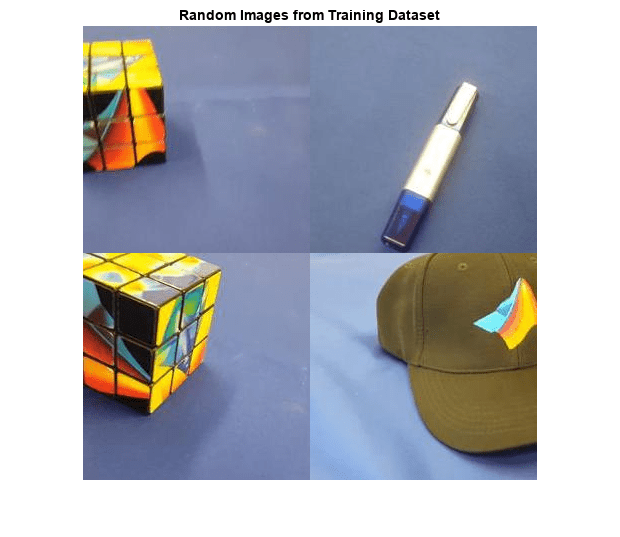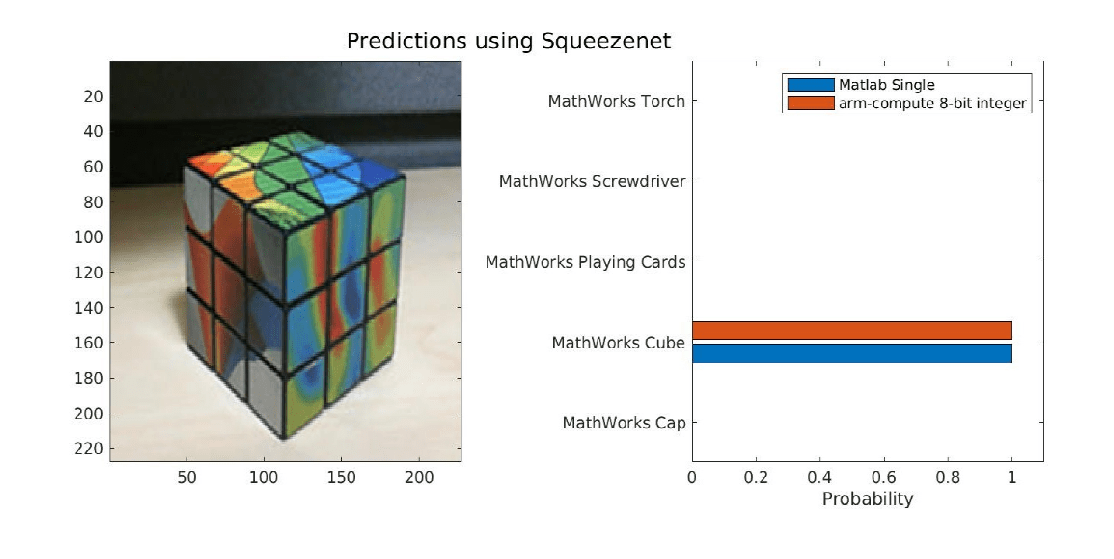# Generate `INT8` Code for Deep Learning Network on Raspberry Pi

Deep learning uses neural network architectures that contain many processing layers, including convolutional layers. Deep learning models typically work on large sets of labeled data. Performing inference on these models is computationally intensive, consuming significant amount of memory. Neural networks use memory to store input data, parameters (weights), and activations from each layer as the input propagates through the network. Deep Neural networks trained in MATLAB use single-precision floating point data types. Even networks that are small in size require a considerable amount of memory and hardware to perform these floating-point arithmetic operations. These restrictions can inhibit deployment of deep learning models to devices that have low computational power and smaller memory resources. By using a lower precision to store the weights and activations, you can reduce the memory requirements of the network.

You can use Deep Learning Toolbox in tandem with the Deep Learning Toolbox Model Quantization Library support package to reduce the memory footprint of a deep neural network by quantizing the weights, biases, and activations of convolution layers to 8-bit scaled integer data types. The quantization is performed by providing the calibration result file produced by the `calibrate` (Deep Learning Toolbox) function to the `codegen` command. Then, you can use MATLAB Coder™ to generate optimized code for the this network. The generated code takes advantage of ARM® processor SIMD by using the ARM Compute library. The generated code can be integrated into your project as source code, static or dynamic libraries, or executables that you can deploy to a variety of ARM CPU platforms such as Raspberry Pi™.

This example shows how to generate C++ code for a convolutional neural network that uses the ARM Compute Library and performs inference computations in 8-bit integers.

This example is not supported for MATLAB Online.

Third-Party Prerequisites

### Example: Classify Images Using SqueezeNet

In this example, you use MATLAB Coder to generate optmized C++ code for a deep convolutional neural network and classify an image. The generated code performs inference computation using 8-bit integer data type for the convolution layer. The example uses the pretrained `squeezenet` (Deep Learning Toolbox) convolutional neural network.

SqueezeNet has been trained on the ImageNet dataset containing images of 1000 object categories. The network has learned rich feature representations for a wide range of images. The network takes an image as input and outputs a label for the object in the image together with the probabilities for each of the object categories.

This example consists of four steps:

1. Modify the SqueezeNet neural network to classify a smaller subset of images containing five object categories using transfer learning.

2. Use the `calibrate` function to exercise the network with sample inputs and collect range information to produce a calibration result file.

3. Generate optimized code for the network by using the codegen command and the calibration result file. The generated code runs on Raspberry Pi target via PIL execution.

4. Execute the generated PIL MEX on Raspberry Pi.

### Transfer Learning Using SqueezeNet

To perform classification on a new set of images, you must fine-tune a pretrained SqueezeNet convolutional neural network by using transfer learning. In transfer learning, you take a pretrained network and use it as a starting point to learn a new task. Fine-tuning a network by using transfer learning is usually much faster and easier than training a network with randomly initialized weights from scratch. You can quickly transfer learned features to a new task by using a smaller number of training images.

Unzip and load the new images as an image datastore. The `imageDatastore` function automatically labels the images based on folder names and stores the data as an `ImageDatastore` object. An image datastore enables you to store large image data, including data that does not fit in memory, and efficiently read batches of images during training of a convolutional neural network.

```unzip('MerchData.zip'); imds = imageDatastore('MerchData', ... 'IncludeSubfolders',true, ... 'LabelSource','foldernames'); [imdsTrain,imdsValidation] = splitEachLabel(imds,0.7,'randomized'); numTrainImages = numel(imdsTrain.Labels); idx = randperm(numTrainImages,4); img = imtile(imds, 'Frames', idx); figure imshow(img) title('Random Images from Training Dataset');````net=squeezenet;`

The object `net` contains the `DAGNetwork` object. The first layer is the image input layer that accepts input images of size 227-by-227-by-3, where 3 is the number of color channels. Use the `analyzeNetwork` (Deep Learning Toolbox) function to display an interactive visualization of the network architecture, to detect errors and issues in the network, and to display detailed information about the network layers. The layer information includes the sizes of layer activations and learnable parameters, the total number of learnable parameters, and the sizes of state parameters of recurrent layers.

```inputSize = net.Layers(1).InputSize; analyzeNetwork(net);```

#### Replace Final Layers

The convolutional layers of the network extract image features that the last learnable layer and the final classification layer use to classify the input image. These two layers, `'conv10'` and` ``'ClassificationLayer_predictions'` in SqueezeNet, contain information about how to combine the features that the network extracts into class probabilities, a loss value, and predicted labels.

To retrain a pretrained network to classify new images, replace these two layers with new layers adapted to the new data set. You can do this manually or use the helper function `findLayersToReplace` to find these layers automatically.` `

This is the `findLayersToReplace` helper function:

`type findLayersToReplace.m`
```function [learnableLayer,classLayer] = findLayersToReplace(lgraph) % findLayersToReplace(lgraph) finds the single classification layer and the % preceding learnable (fully connected or convolutional) layer of the layer % graph lgraph. % Copyright 2021 The MathWorks, Inc. if ~isa(lgraph,'nnet.cnn.LayerGraph') error('Argument must be a LayerGraph object.') end % Get source, destination, and layer names. src = string(lgraph.Connections.Source); dst = string(lgraph.Connections.Destination); layerNames = string({lgraph.Layers.Name}'); % Find the classification layer. The layer graph must have a single % classification layer. isClassificationLayer = arrayfun(@(l) ... (isa(l,'nnet.cnn.layer.ClassificationOutputLayer')|isa(l,'nnet.layer.ClassificationLayer')), ... lgraph.Layers); if sum(isClassificationLayer) ~= 1 error('Layer graph must have a single classification layer.') end classLayer = lgraph.Layers(isClassificationLayer); % Traverse the layer graph in reverse starting from the classification % layer. If the network branches, throw an error. currentLayerIdx = find(isClassificationLayer); while true if numel(currentLayerIdx) ~= 1 error('Layer graph must have a single learnable layer preceding the classification layer.') end currentLayerType = class(lgraph.Layers(currentLayerIdx)); isLearnableLayer = ismember(currentLayerType, ... ['nnet.cnn.layer.FullyConnectedLayer','nnet.cnn.layer.Convolution2DLayer']); if isLearnableLayer learnableLayer = lgraph.Layers(currentLayerIdx); return end currentDstIdx = find(layerNames(currentLayerIdx) == dst); currentLayerIdx = find(src(currentDstIdx) == layerNames); %#ok<FNDSB> end end ```

To use this function to replace the final layers, run these commands:

```lgraph = layerGraph(net); [learnableLayer,classLayer] = findLayersToReplace(lgraph); numClasses = numel(categories(imdsTrain.Labels)); newConvLayer = convolution2dLayer([1, 1],numClasses,'WeightLearnRateFactor',... 10,'BiasLearnRateFactor',10,"Name",'new_conv'); lgraph = replaceLayer(lgraph,'conv10',newConvLayer); newClassificatonLayer = classificationLayer('Name','new_classoutput'); lgraph = replaceLayer(lgraph,'ClassificationLayer_predictions',newClassificatonLayer);```

#### Train Network

The network requires all input images to have the size 227-by-227-by-3, but each image in the image datastores has a differnet size. Use an augmented image datastore to automatically resize the training images. Specify these additional augmentation operations to be performed on the training images: randomly flip the training images about the vertical axis, and randomly translate them up to 30 pixels horizontally and vertically. Data augmentation helps prevent the network from over-fitting and memorizing the exact details of the training images.

```pixelRange = [-30 30]; imageAugmenter = imageDataAugmenter( ... 'RandXReflection',true, ... 'RandXTranslation',pixelRange, ... 'RandYTranslation',pixelRange); augimdsTrain = augmentedImageDatastore(inputSize(1:2),imdsTrain, ... 'DataAugmentation',imageAugmenter);```

To automatically resize the validation images without performing further data augmentation, use an augmented image datastore without specifying any additional preprocessing operations.

`augimdsValidation = augmentedImageDatastore(inputSize(1:2),imdsValidation);`

Specify the training options. For transfer learning, keep the features from the early layers of the pretrained network (the transferred layer weights). To slow down learning in the transferred layers, set the initial learning rate to a small value. In the previous step, you increased the learning rate factors for the convolutional layer to speed up learning in the new final layers. This combination of learning rate settings results in fast learning only in the new layers and slower learning in the other layers. When performing transfer learning, you do not need to train for as many epochs. An epoch is a full training cycle on the entire training data set. Specify the mini-batch size to be 11 so that in each epoch you consider all of the data. During training, the software validates the network after every `ValidationFrequency` iterations.

```options = trainingOptions('sgdm', ... 'MiniBatchSize',11, ... 'MaxEpochs',7, ... 'InitialLearnRate',2e-4, ... 'Shuffle','every-epoch', ... 'ValidationData',augimdsValidation, ... 'ValidationFrequency',3, ... 'Verbose',false);```

Train the network that consists of the transferred and new layers.

```netTransfer = trainNetwork(augimdsTrain,lgraph,options); classNames = netTransfer.Layers(end).Classes; save('mySqueezenet.mat','netTransfer');```

### Generate Calibration Result File for the Network

Create a `dlquantizer` object and specify the network. Note that code generation does not support quantized deep neural networks produced by the `quantize` (Deep Learning Toolbox) function.

`quantObj = dlquantizer(netTransfer, 'ExecutionEnvironment', 'CPU');`

Use the `calibrate` function to exercise the network with sample inputs and collect range information. The `calibrate` function exercises the network and collects the dynamic ranges of the weights and biases in the convolution and fully connected layers of the network and the dynamic ranges of the activations in all layers of the network. The function returns a table. Each row of the table contains range information for a learnable parameter of the optimized network.

```calResults = quantObj.calibrate(augimdsTrain); save('squeezenetCalResults.mat','calResults'); save('squeezenetQuantObj.mat','quantObj');```

### Generate PIL MEX Function

In this example, you generate code for the entry-point function `predict_int8`. This function uses the `coder.loadDeepLearningNetwork` function to load a deep learning model and to construct and set up a CNN class. Then the entry-point function predicts the responses by using the `predict` (Deep Learning Toolbox) function.

`type predict_int8.m`
```function out = predict_int8(netFile, in) persistent mynet; if isempty(mynet) mynet = coder.loadDeepLearningNetwork(netFile); end out = predict(mynet,in); end ```

To generate a PIL MEX function, create a code configuration object for a static library and set the verification mode to `'PIL'`. Set the target language to C++.

``` cfg = coder.config('lib', 'ecoder', true); cfg.VerificationMode = 'PIL'; cfg.TargetLang = 'C++';```

Create a deep learning configuration object for the ARM Compute library. Specify the library version and arm architecture. For this example, suppose that the ARM Compute Library in the Raspberry Pi hardware is version 20.02.1.

``` dlcfg = coder.DeepLearningConfig('arm-compute'); dlcfg.ArmComputeVersion = '20.02.1'; dlcfg.ArmArchitecture = 'armv7';```

Set the properties of `dlcfg` to generate code for low precision/INT8 inference.

``` dlcfg.CalibrationResultFile = 'squeezenetQuantObj.mat'; dlcfg.DataType = 'int8';```

6. Set the `DeepLearningConfig` property of `cfg` to `dlcfg`.

` cfg.DeepLearningConfig = dlcfg;`

7. Use the MATLAB Support Package for Raspberry Pi function, `raspi`, to create a connection to the Raspberry Pi. In the following code, replace:

• `raspiname` with the name of your Raspberry Pi

• `username` with your user name

• `password` with your password

`% r = raspi('raspiname','username','password');`

8. Create a `coder.Hardware` object for Raspberry Pi and attach it to the code generation configuration object.

```% hw = coder.hardware('Raspberry Pi'); % cfg.Hardware = hw;```

9. Generate a PIL MEX function by using the `codegen` command

`% codegen -config cfg predict_int8 -args {coder.Constant('mySqueezenet.mat'), ones(227,227,3,'uint8')}`

### Run Generated PIL MEX Function on Raspberry Pi

Input image is expected to be of same size as the input size of the network. Read the image that you want to classify and resize it to the input size of the network. This resizing slightly changes the aspect ratio of the image.

```% testImage = imread("MerchDataTest.jpg"); % testImage = imresize(testImage,inputSize(1:2));```

To compare the predictions of the Deep Learning Toolbox `predict` function and the generared PIL MEX function `predict_int8_pil`, call both these funcions on the input image separately.

```% predictScores(:,1) = predict(netTransfer,testImage)'; % predictScores(:,2) = predict_int8_pil('mySqueezenet.mat',testImage)';```

Display the predicted labels and their associated probabilities as a histogram.

```% h = figure; % h.Position(3) = 2*h.Position(3); % ax1 = subplot(1,2,1); % ax2 = subplot(1,2,2); % image(ax1,testImage); % barh(ax2,predictScores) % xlabel(ax2,'Probability') % yticklabels(ax2,classNames) % ax2.XLim = [0 1.1]; % ax2.YAxisLocation = 'left'; % legend('Matlab Single','arm-compute 8-bit integer'); % sgtitle('Predictions using Squeezenet') % saveas(gcf,'SqueeznetPredictionComparison.jpg'); % close(gcf); imshow('SqueeznetPredictionComparison.jpg');```